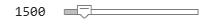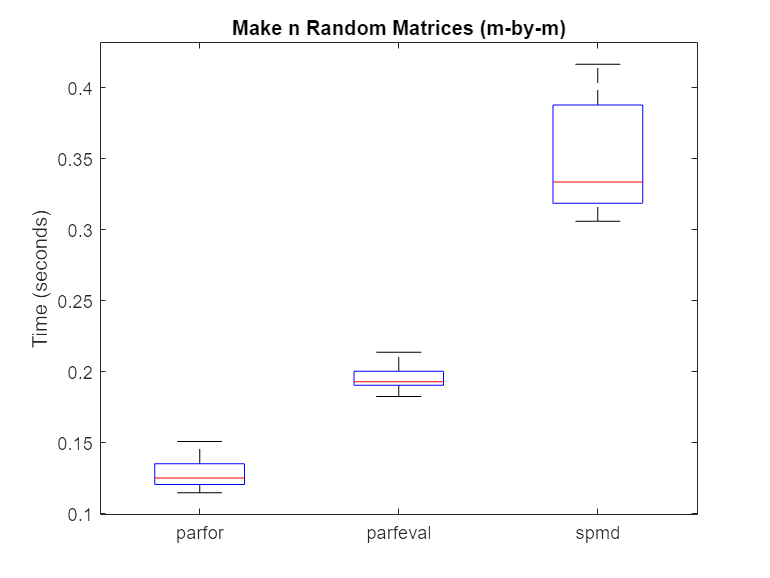## Choose Between `spmd`, `parfor`, and `parfeval`

To run computations in parallel, you can use `spmd`, `parfor`, `parfeval`, or `parfevalOnAll`. Each construct relies on different parallel programming concepts.

### When to Use `parfor`

A `parfor`-loop can be useful if you have a `for`-loop with slow loop iterations or many loop iterations. Each iteration must be independent of all others. For more help in deciding when to use parfor, see Decide When to Use parfor.

### When to Use `spmd`

#### Run Communicating Parallel Code

Use `spmd` if you require fine-grained worker-to-worker communication and collaboration between workers during a computation. `parfor`, `parfeval`, and `parfevalOnAll` do not allow communication between workers. Computations with `spmd` can involve communication between workers using the `spmdSend`, `spmdReceive`, and `spmdSendReceive` functions.

If you are unsure, ask yourself the following: within my parallel code, can each computation be completed without any communication between workers? If yes, use `parfor` or `parfeval`. Otherwise, use `spmd`.

#### Run Parallel and Customized Code on Distributed Arrays

Use `spmd` if your computations involve large arrays distributed across workers. You can perform simultaneous calculations on all workers or perform customized calculations on specific workers.

When workers run an `spmd` block, each worker is assigned a unique index, the `spmdIndex`. This lets you specify code to be run only on certain workers, and target sections of distributed arrays.

#### Synchronous and Asynchronous Work

When choosing between `parfor`, `parfeval`, and `spmd`, consider whether your calculation requires synchronization with the client.

`parfor` and `spmd` require synchronization, and therefore block you from running any new computations on the MATLAB® client. `parfeval` does not require synchronization, so you can continue to use the client.

#### Use Other Functional Capabilities

`spmd` and `parfeval` have other capabilities you can use after you have submitted your computations.

• With `spmd`, you can collect results computed inside the `spmd` statement without transferring the results to the client. Access the values of the variables assigned inside the `spmd` statement as `Composite` objects from the client. For more information, see Access Worker Variables with Composites.

• When you submit a `parfeval` task, MATLAB schedules the task to run asynchronously and returns `Future` objects before the submitted task finishes running. A `Future` object represents the task that MATLAB has scheduled. You can interact with `Future` objects in different ways:

• Use `fetchNext` to retrieve results as they become available or check if results are ready.

• Stop `parfeval` calculations from running using the `cancel` function.

• Use `Future` objects in other computation on the client.

### Compare Performance of `parfor`, `parfeval`, and `spmd`

Using `spmd` can be slower or faster than using `parfor`-loops or `parfeval`, depending on the type of computation. Overhead affects the relative performance of `parfor`-loops, `parfeval`, and `spmd`.

For a set of tasks, `parfor` and `parfeval` typically perform better than `spmd` under these conditions:

• The computational time taken per task is not deterministic.

• The computational time taken per task is not uniform.

• The data returned from each task is small.

Use `parfeval` when:

• You want to run computations in the background.

In this example, you examine the speed at which the software performs matrix operations when using a `parfor`-loop, `parfeval`, and `spmd`.

First, create a parallel pool of process workers `p`.

`p = parpool("Processes");`
```Starting parallel pool (parpool) using the 'Processes' profile ... Connected to parallel pool with 6 workers. ```

Compute Random Matrices

Examine the speed at which the software can generate random matrices by using a `parfor`-loop, `parfeval`, and `spmd`. Set the number of trials (`n`) and the matrix size (for an `m`-by-`m` matrix). Increasing the number of trials improves the statistics used in later analysis, but does not affect the calculation itself.

```m =1500; n =20;```

Then, use a `parfor`-loop to execute `rand(m)` once for each worker. Time each of the `n` trials.

```parforTime = zeros(n,1); for i = 1:n tic; mats = cell(1,p.NumWorkers); parfor N = 1:p.NumWorkers mats{N} = rand(m); end parforTime(i) = toc; end```

Next, use `parfeval` to execute `rand(m)` once for each worker. Time each of the `n` trials.

```parfevalTime = zeros(n,1); for i = 1:n tic; f(1:p.NumWorkers) = parallel.FevalFuture; for N = 1:p.NumWorkers f(N) = parfeval(@rand,1,m); end mats = fetchOutputs(f); parfevalTime(i) = toc; clear f end```

Finally, use `spmd` to execute `rand(m)` once for each worker. You can use `spmdCat` to concatenate the values of `mat` on each worker into array `mats` and store it on worker 1. For details on workers and how to execute commands on them with `spmd`, see Run Single Programs on Multiple Data Sets. Time each of the `n` trials.

```spmdTime = zeros(n,1); for i = 1:n tic; spmd mat = rand(m); mats = spmdCat({mat}, 1, 1); end allMats = mats{1}; spmdTime(i) = toc; end```

Use `rmoutliers` to remove the outliers from each of the trials. Then, use `boxplot` to compare the times.

```% Hide outliers boxData = rmoutliers([parforTime parfevalTime spmdTime]); % Plot data boxplot(boxData, 'labels',{'parfor','parfeval','spmd'}, 'Symbol','') ylabel('Time (seconds)') title('Make n Random Matrices (m-by-m)')```Typically, `spmd` requires more overhead per evaluation than `parfor` or `parfeval`. Therefore, in this case, using a `parfor`-loop or `parfeval` is more efficient.

Compute Sum of Random Matrices

Next, compute the sum of random matrices. You can do this by using a reduction variable with a `parfor`-loop, a sum after computations with `parfeval`, or `spmdPlus` with `spmd`. Again, set the number of trials (`n`) and the matrix size (for an `m`-by-`m` matrix).

```m =1500; n =20;```

Then, use a `parfor`-loop to execute `rand(m)` once for each worker. Compute the sum with a reduction variable. Time each of the `n` trials.

```parforTime = zeros(n,1); for i = 1:n tic; result = 0; parfor N = 1:p.NumWorkers result = result + rand(m); end parforTime(i) = toc; end```

Next, use `parfeval` to execute `rand(m)` once for each worker. Use `fetchOutputs` to fetch all of the matrices, then use `sum`. Time each of the `n` trials.

```parfevalTime = zeros(n,1); for i = 1:n tic; f(1:p.NumWorkers) = parallel.FevalFuture; for N = 1:p.NumWorkers f(N) = parfeval(@rand,1,m); end result = sum(fetchOutputs(f)); parfevalTime(i) = toc; clear f end```

Finally, use `spmd` to execute `rand(m)` once for each worker. Use `spmdPlus` to sum all of the matrices. To send the result only to the first worker, set the optional target worker argument to `1`. Time each of the `n` trials.

```spmdTime = zeros(n,1); for i = 1:n tic; spmd r = spmdPlus(rand(m), 1); end result = r{1}; spmdTime(i) = toc; end```

Use `rmoutliers` to remove the outliers from each of the trials. Then, use `boxplot` to compare the times.

```% Hide outliers boxData = rmoutliers([parforTime parfevalTime spmdTime]); % Plot data boxplot(boxData, 'labels',{'parfor','parfeval','spmd'}, 'Symbol','') ylabel('Time (seconds)') title('Sum of n Random Matrices (m-by-m)')```For this calculation, `spmd` is faster than a `parfor`-loop or `parfeval`. When you use reduction variables in a `parfor`-loop, each worker performs a local reduction before sending its partial result back to the client to compute the final result.

By contrast, `spmd` calls `spmdPlus` only once to do a global reduction operation, requiring less overhead. As such, the overhead for the reduction part of the calculation is $O\left({n}^{2}\right)$ for `spmd`, and $O\left(m{n}^{2}\right)$ for `parfor`.